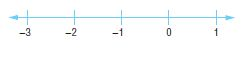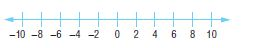Homework Explained - Math Practice 101Dear guest, you are not a registered member. As a guest, you only have read-only access to our books, tests and other practice materials.

As a registered member you can:

Registration is free and doesn't require any type of payment information. Click here to Register.
Go to page:
Chapter 3: Integers; Lesson 1: Integers and Absolute Value

### Independent Practice

Write an integer for each situation.

• Question 1

a profit of $9 • $
• Question 2

a bank withdrawal of $50 • $
• Question 3

53°C below zero

•  $$^o\text{C}$$
• Question 4

7 inches more than normal

Graph each set of integers on a number line.

• Question 5

{0, 1, -3}• Question 6

{-5, -1, 10, -9}Evaluate each expression.

•  $$|10| =$$
•  $$|-7| - 5 =$$
•  $$1+ |7|=$$
• Question 10

The number of yards a football team moves on the field can be represented using the expression |8| + |-4|. How many yards does the football team move?

•  yards
• Question 11

In golf, scores are often written in relationship to par, the average score for a round at a certain course. Write an integer to represent a score that is 7 under par.Yes, email page to my online tutor. (if you didn't add a tutor yet, you can add one here)